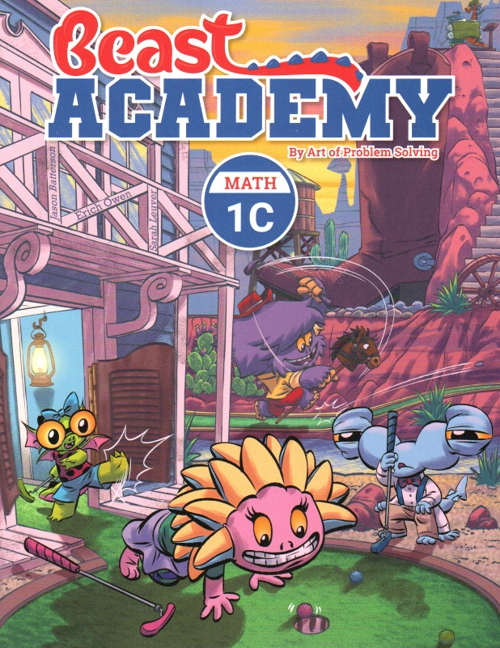Art of Problem Solving Beast Academy 1C Guide and Practice \$27 : Addition & Subtraction: Strategies review, using the number line, distance on the number line, adding and subtracting tens, adding by place value. Comparing: Ordering numbers using place value, comparing expressions, finding sums and differences that are equal, compensation strategies. Patterns: Completing patterns that use shapes and numbers, skip-counting, and special patterns including the Fibonacci sequence, perfect squares, and triangular numbers.